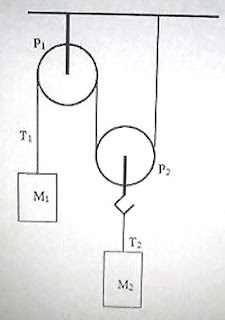## Pages

`“Life is like riding a bicycle.  To keep your balance you must keep moving.”–Albert Einstein`

## Thursday, June 18, 2009

### AP Physics B&C – Answer to Free Response Practice Question on Newton’s Laws

In the post dated 15th June 2009, the following free-response question for practice was given to you:In the adjoining figure P1 and P2 are light frictionless pulleys. P1 is fixed and P2 is movable. The strings used are inextensible and of negligible mass. The fixed pulley P1 carries a mass M1 and the movable pulley P2 carries a mass M2 which has one and a half times the mass of M1. Now, answer the following questions:

(a) How is the tension T1 in the string attached to the mass M1 related to the tension T2 in the string attached to the mass M2?

(b) How is the magnitude of acceleration a1 of the mass M1 related to the magnitude of acceleration a2 of the mass M2?

(c) From the following options regarding the motion of the mass M1, select the correct one by putting a tick (√) mark:

Mass M1 will be accelerated upwards ______

Mass M1 will be accelerated downwards ______

Mass M1 will move with uniform velocity ______

(d) Determine the accelerations a1 and a2 in of the masses M1 and M2 respectively.

(e) Determine the tensions T1 and T2 in the strings attached to the masses M1 and M2 respectively.

(a) The forces acting on the system of masses are shown in the figure. The string passing over the pulleys P1 and P2 has the same tension T1 everywhere. Since the tension T2 in the string connected to the mass M2 is produced because of the tensions T1 and T1 it follows that

T2 = T1 + T1 = 2 T1

(b) When the mass M1 moves through a distance x, the mass M2 moves through a distance x/2 only. Therefore the magnitude of acceleration a1 of the mass M1 is twice the magnitude of acceleration a2 of the mass M2:

a1 = 2a2

(c) If the masses were such that the system were stationary we would have

M1g = T1 and M2g = T2 = 2 T1

From the above equations M2 = 2M1.

Since it is given in the question that M2 = 1.5 M1, it follows that the system cannot be stationary. Evidently the mass M1 will be accelerated downwards (and M2 will be accelerated upwards).

(d) The net force acting on M1 = M1g T1

Therefore, M1g T1 = M1a1 ………………(i)

Similarly, considering mass M2 we obtain

2T1 M2g = M2 a1/2 …………….(ii)

[We have used T2 = 2T1 and a2 = a1/2].

Multiplying Eq.(i) by 2 and adding to Eq.(ii) we obtain

2M1g M2g = a1[2M1 + (M2/2)]

This gives

a1 = 2g(2M1M2) /(4M1 + M2)

This is directed downwards.

Since a2 = a1/2 we have

a2 = g(2M1M2) /(4M1 + M2), which is directed upwards.

(e) Substituting for a1 in Eq.(i),

T1 = M1(g a1) = M1[g {2g(2M1M2) /(4M1 + M2)}]

Or, T1 = M1[(4M1 + M2)g 2g(2M1M2)] /(4M1 + M2)

This simplifies to T1 = 3M1M2g /(4M1 + M2)

Since T2 = 2T1 we obtain

T2 = 6M1M2g /(4M1 + M2)